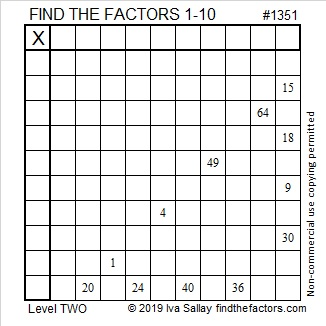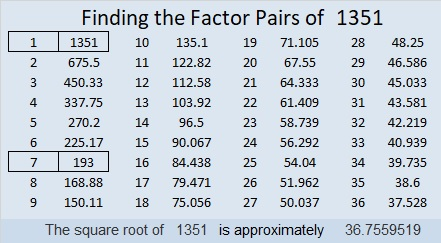# 1351 and a Level 2 Smiley Face

You will smile a great big smile once you solve this puzzle!Print the puzzles or type the solution in this excel file: 10 Factors 1347-1356

If you want a little help solving the puzzle, I explain how to do it in this video:

Here are some facts about the puzzle number 1351:

• 1351 is a composite number.
• Prime factorization: 1351 = 7 × 193
• 1351 has no exponents greater than 1 in its prime factorization, so √1351 cannot be simplified.
• The exponents in the prime factorization are 1, and 1. Adding one to each exponent and multiplying we get (1 + 1)(1 + 1) = 2 × 2 = 4. Therefore 1351 has exactly 4 factors.
• The factors of 1351 are outlined with their factor pair partners in the graphic below.1351 is the hypotenuse of a Pythagorean triple:
665-1176-1351 which is 7 times (95-168-193)

This site uses Akismet to reduce spam. Learn how your comment data is processed.